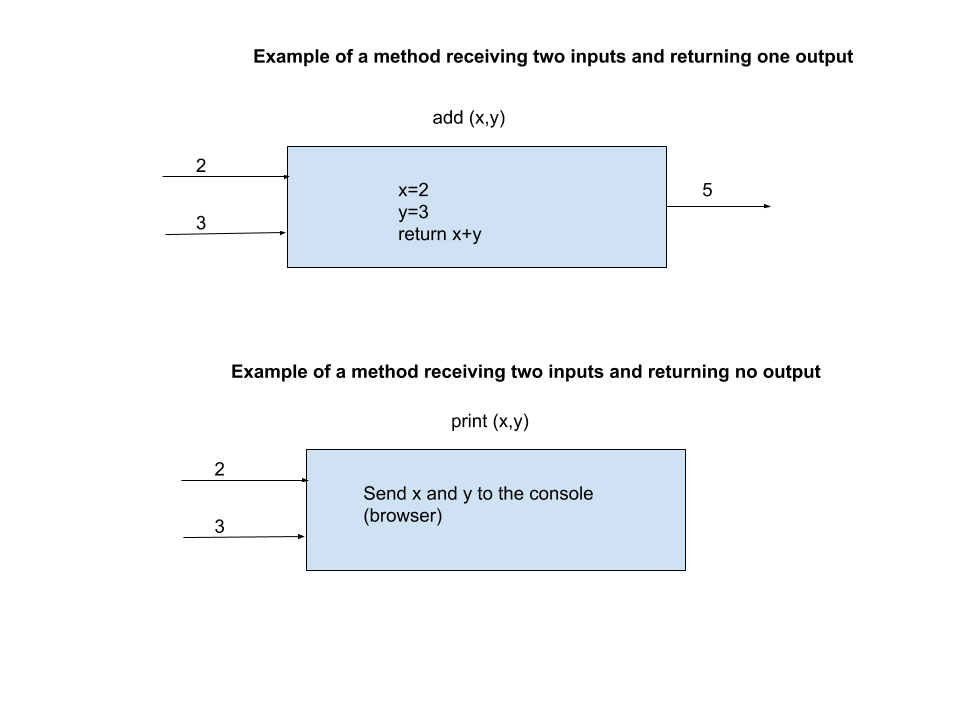# Recap

### What is a function?

A function is a set of statements that take zero to more inputs, do some computation and produces zero to more outputs. You send values to a function between a pair of parenthesis. If the function returns something then you receive that by declaring a variable.### What is a module?

A module is nothing but a Python program, which can be used in another program. There are hundreds of modules written by many different programmers solving some specific problems and typically any decent Python program will use many different modules.

# Titanic workshop

``````
import pandas as pd
import seaborn as sns

``````

In the above code block, we use two different modules, pandas and seaborn. Pandas is renamed as 'pd', and Seaborn is renamed as 'sns'. Both these have many convenient functions which we will use.

On 'sns' you use the 'load_dataset' function by giving the name of the dataset to load. This module already has titanic data inside of it. So it is able to give us back a structure called 'dataframe' which we have saved in a variable declared as 'titanic_df'. a dataframe is a table like structure as you can see.

On this dataframe, we can invoke many convenient functions. One of them is a head function which takes in a number 'n' as input and then gives back the top 'n' records as the output.

### To get the entire column values

You can get a specific column values, you simply enclose the column name in single or double quote inside a pair of square bracket:

``````
titanic_df['age']
``````

NaN stands for Not a Number which means it either is empty or there is some invalid value.

### To get the mean use mean() function

To find the mean age of all passengers in Titanic ship, you would use:

``````
mean_age = titanic_df['age'].mean()
print(mean_age)
``````

There are more such functions, median, max, min.

### To find the 'n' number of largest values you use 'nlargest'

``````
titanic_df['age'].nlargest(4)
``````

In this you pass in any number as input and it will return that many values of the age column sorted from largest to smallest.

Complementary to 'nlargest' is 'nsmallest'.

### value_counts() function

"value_counts()" function returns a structure which has the total count of the unique value in the column. When you apply this against a categorical column, you will see the total count against each category.

``````
titanic_df['embark_town'].value_counts()
``````

### plot function

You use the plot function to plot graphs. The two charts we will apply are 'histogram' and 'bar'

For 'age' column we will apply histogram and for the value_counts of the categorial values, we will apply 'bar'

``````
titanic_df['age'].plot(kind='hist')
``````

### So what is the input for the plot function now? You can also add another input to get the title and that is to use the 'title' key. Can you do that?

``````
titanic_df['embark_town'].value_counts().plot(kind='bar')
``````